Courses

# Test: Refining of Crude Metals

## 25 Questions MCQ Test Chemistry Class 12 | Test: Refining of Crude Metals

Description
This mock test of Test: Refining of Crude Metals for JEE helps you for every JEE entrance exam. This contains 25 Multiple Choice Questions for JEE Test: Refining of Crude Metals (mcq) to study with solutions a complete question bank. The solved questions answers in this Test: Refining of Crude Metals quiz give you a good mix of easy questions and tough questions. JEE students definitely take this Test: Refining of Crude Metals exercise for a better result in the exam. You can find other Test: Refining of Crude Metals extra questions, long questions & short questions for JEE on EduRev as well by searching above.
QUESTION: 1

### Only One Option Correct Type Direction (Q. Nos. 1-10) This section contains 10 multiple choice questions. Each question has four choices (a), (b), (c) and (d), out of which ONLY ONE is correc Q. Poling process is used

Solution:

Poling (metallurgy) is a method employed in the purification of copper which contains cuprous oxide as an impurity.

QUESTION: 2

### In the electrolytic refining of zinc

Solution:

In this method, the impure metal is converted into a block which forms the anode while cathode is made up of a pure strip of the same metal. These electrodes are suspended in an electrolyte which is the solution of a soluble salt of the metal usually double salt of the metal.

QUESTION: 3

### The super pure silicon can be obtained by

Solution:

Zone refining This method is employed for preparing extremely pure metals. Germanium, silicon, boron, gallium and indium are purified by this method.
This method is based upon the principle that when a molten solution of the impure metal is allowed to cool the pure metal crystallises out while the impurities remain in the melt.

QUESTION: 4

Purification of aluminium is called

Solution:

The Hoope's process is a metallurgical process used to obtain the aluminium metal of very high purity. It gives about 99.99% pure aluminium.

QUESTION: 5

The processes used in the refining of aluminium and zinc metals respectively are

Solution:

Cupellation This method is applied when the impure metal contains impurities of other metals which form volatile oxides. Distillation process This method is employed for purification of volatile metals like zinc, mercury, cadmium etc. The impure metal is heated in an iron retort and the vapours are condensed in separate receivers.

QUESTION: 6

van Arkel method of purification of metals involves converting the metal to

Solution:

van Arkel method This method is used for preparation of ultra pure metals such as titanium and zirconium which are used in space technology. In this method, zirconium is heated in iodine vapours at about 870 K to form unstable volatile zirconium tetraiodide which when heated over a tungsten filament at 2075 K decompose to give pure zirconium.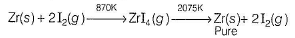QUESTION: 7

The most electropositive metals are extracted from their ores by

Solution:

The extraction of highly electropositive metals is carried out by electrolysis of their oxides, hydroxides etc., in fused state because their compounds are not reduced by ordinary reducing agents.

QUESTION: 8

Which method of purification is represented by the following equation?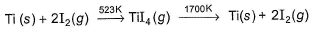Solution:

van Arkel method This method is used in the production of ultra-pure titanium and zirconium. It primarily involves the formation of the metal iodides and their subsequent decompositon to yield pure metal.

QUESTION: 9

Which of the following process is used in the extractive metallurgy of magnesium?

Solution:

Since Mg is highly electropositive its extractive metallurgy is carried out by fused salt electrolysis.

QUESTION: 10

In the electrolytic refining of copper using CuSO4 as electrolyte the anod reaction is

Solution:

The process takes place are under.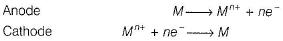The net result is the transfer of pure metal from the anode to the cathode,

*Multiple options can be correct
QUESTION: 11

One or More than One Options Correct Type

Direction (Q. Nos. 11-15) This section contains 5 multiple choice questions. Each question has four choices (a), (b), (c) and (d), out of which ONE or MORE THAN ONE are correct.

Q.

Which of the following is related to metal purification?

Solution:

Mac Arthur Forrest process It is a metallurgical technique for extracting gold from low grade ore by converting the gold to a water soluble coordination complex. It is the most commonly used process for gold extraction.

*Multiple options can be correct
QUESTION: 12

Which of the following are correctly matched?

Solution:

Mond process is used for refining of nickel. When impure nickel is heated in a current of CO at 330-350 K. It forms volatile nickel carbonyl leaving behind the impurities.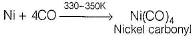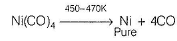*Multiple options can be correct
QUESTION: 13

Regarding blister copper incorrect statements are

Solution:

The self reduction of the oxide and sulphide occurs, giving impure, blister copper which is 98-99% pure.
Cu2S + 2Cu2O → 6Cu + SO2
The blister copper is cast into blocks and refined by electrolysis using Cu electrodes with an electrolyte of dilute H2SO4 and CuSO4.

*Multiple options can be correct
QUESTION: 14

Regarding electrolytic refining of silver which is correct?

Solution:

Ag can be refined by electrolysis of AgNO3 (containing HNO3) using impure Ag as anode and pure Ag as cathode.

*Multiple options can be correct
QUESTION: 15

In the chromatography method of purification of metals the adsorbent used in the column

Solution:

Alumina (Al2O3) and silica gel used as adsorbet in column chromatography.

QUESTION: 16

Comprehension Type

Direction (Q. Nos. 16 and 17) This section contains a paragraph, describing theory, experiments, data, etc. Two questions related to the paragraph have been given. Each question has only one correct answer among the four given options (a), (b), (c) and (d).

Passage

Impure metals are purified by different methods in order to remove the undesired impurities. These methods depend on the nature of metal and nature of impurities. Liquation is used for purifying metals with low melting points like Bi, Sn, Pb, Hg etc. Distillation is used for volatile metals like Zn, Cd, Hg. Cupellation is used to purify silver metal with lead impurities. Electrolytic refining (Cu, Ag, Mg, Al) Zone refining (Si, Ge, Ga) Mond’s process (Ni) van Arkel process (Zr, Ti).

Q.

In the extraction of nickel by Mond’s process, the metal is obtained by

Solution:

The metal is obtained by thermal decomposition. Impure nickel is heated in a current of CO at 330-350 K. It forms volatile nickel carbonyl leaving behind the impurities.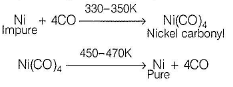QUESTION: 17

Impure metals are purified by different methods in order to remove the undesired impurities. These methods depend on the nature of metal and nature of impurities. Liquation is used for purifying metals with low melting points like Bi, Sn, Pb, Hg etc. Distillation is used for volatile metals like Zn, Cd, Hg. Cupellation is used to purify silver metal with lead impurities. Electrolytic refining (Cu, Ag, Mg, Al) Zone refining (Si, Ge, Ga) Mond’s process (Ni) van Arkel process (Zr, Ti).

Q.

Suggest a method of purification for obtaining highly pure solar grade silicon used as a semiconductor.

Solution:

Zone refining (Fractional crystallisation). This method is employed for preparing extremely pure metals, e.g. germanium, silicon, boron, gallium and indium.

QUESTION: 18

Matching List Type

Direction (Q. No. 18 and 19) Choices for the correct combination of elements from Column I and Column II are given as options (a), (b), (c) and (d), out of which one is correct.

Q.

Match the Column I with Column II and mark the correct option from the codes given below.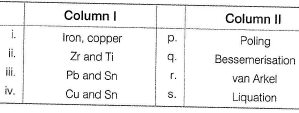Solution:

(i) → (q), (ii) → (r), (iii) → (s), (iv) → (p)

QUESTION: 19

Match the Column I with Column II and mark the correct option from the codes given below.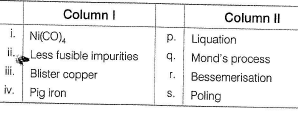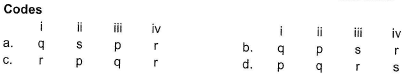Solution:

(i) → (q), (ii) →(p), (iii) → (s), (iv) → (r)

*Answer can only contain numeric values
QUESTION: 20

One Integer Value Correct Type

Direction (Q. Nos. 20-24) This section contains 5 questions. When worked out will result in an integer from 0 to 9 (both inclusive).

Q.

In auto reduction process of copper, the part of cuprous sulphide is oxidised with cuprous oxide to form a free copper metal. The balanced equation is as follows

Cu2S + 2Cu2O → xCu + SO2

The value of x is

Solution: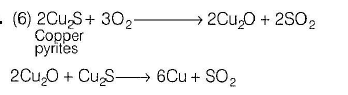*Answer can only contain numeric values
QUESTION: 21

The central atom in the stable volatile complex formed in the Mond’s method has the oxidation number

Solution:

The complex formed in the Mond process is (Ni(CO)4).
Let the oxidation state of N be x.
Therefore, x + 4 (0) = 0
x = 0

*Answer can only contain numeric values
QUESTION: 22

Number of ligands in the stable volatile complex formed during the extraction of Ni by Mond’s process.

Solution:

Nickel is purified by Mond's process.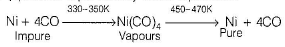*Answer can only contain numeric values
QUESTION: 23

Sea water contains 1297.3g of Mg2+ per metric ton. How much of slaked lime in kg, must be added to 1.0 metric ton of sea water to precipitate all of the Mg2+ ion?

Solution: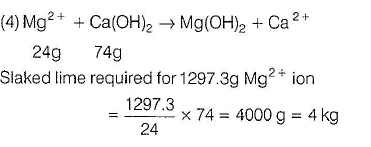*Answer can only contain numeric values
QUESTION: 24

The percentage impurity in 23 carat gold is nearly

Solution:

24 carat gold = 100% pure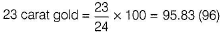Hence, % impurity is nearly 4%.

QUESTION: 25

Statement Type

Direction (Q. No. 25) This section is based on Statement I and Statement II. Select the correct answer from the codes given below.

Q.

Satement I : In electrorefining process cathode is made up of a pure strip of the same metal.

Statement II : Blister copper contains 50% impurities.

Solution:

Blister copper contains 0 - 2 % impurities.